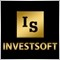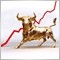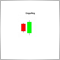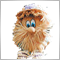70

Hi ...

My code is the following ... and I'm getting an error saying that "array out of range in '1.mq5' (66,33)"

It is the hand-numbered row in the code.

How can I correct this error?

Thanks a lot.

```//+------------------------------------------------------------------+
//|                                                            1.mq5 |
//|                        Copyright 2015, MetaQuotes Software Corp. |
//|                                             https://www.mql5.com |
//+------------------------------------------------------------------+
#property version   "1.00"
#property indicator_chart_window
#property indicator_buffers 2
#property indicator_plots   1
//--- plot Label1
#property indicator_label1  "Label1"
#property indicator_type1   DRAW_COLOR_LINE
#property indicator_style1  STYLE_SOLID
#property indicator_width1  1

input int maPeriod = 10;
input int maShift = 0;
input ENUM_MA_METHOD maMethod = MODE_SMA;
input ENUM_APPLIED_PRICE maPrice = PRICE_CLOSE;

//--- indicator buffers
double         Label1Buffer[];
double         Label1Colors[];

double maBuffer[];
int maHandle;
//+------------------------------------------------------------------+
//| Custom indicator initialization function                         |
//+------------------------------------------------------------------+
int OnInit()
{
//--- indicator buffers mapping
SetIndexBuffer(0,Label1Buffer,INDICATOR_DATA);
SetIndexBuffer(1,Label1Colors,INDICATOR_COLOR_INDEX);

ArraySetAsSeries(Label1Buffer,true);
ArraySetAsSeries(Label1Colors,true);

ArraySetAsSeries(maBuffer, true);
maHandle = iMA(_Symbol,0,maPeriod,maShift,maMethod,maPrice);
//---
return(INIT_SUCCEEDED);
}
//+------------------------------------------------------------------+
//| Custom indicator iteration function                              |
//+------------------------------------------------------------------+
int OnCalculate(const int rates_total,
const int prev_calculated,
const int begin,
const double &price[])
{
//---
int bars = rates_total - 1;
if(prev_calculated > 0)
{
bars = rates_total - prev_calculated;
}

CopyBuffer(maHandle,0,0,bars,maBuffer);

for(int i = bars; i >= 0; i--)
{
66    Label1Buffer[i] = maBuffer[i];
if(((maBuffer[i] - maBuffer[i + 1]) / maBuffer[i + 1]) < ((maBuffer[i + 1] - maBuffer[i + 2]) / maBuffer[i + 2])) Label1Colors[i] = 0;
if(maBuffer[i] < maBuffer[i + 1]) Label1Colors[i] = 1;
if(price[i] < maBuffer[i]) Label1Colors[i] = 2;
if(((maBuffer[i] - maBuffer[i + 1]) / maBuffer[i + 1]) > ((maBuffer[i + 1] - maBuffer[i + 2]) / maBuffer[i + 2])) Label1Colors[i] = 6;
if(maBuffer[i] > maBuffer[i + 1]) Label1Colors[i] = 5;
if(price[i] > maBuffer[i]) Label1Colors[i] = 4;
}
//--- return value of prev_calculated for next call
return(rates_total);
}
//+------------------------------------------------------------------+```25216

OzanK:

Hi ...

My code is the following ... and I'm getting an error saying that "array out of range in '1.mq5' (66,33)"

It is the hand-numbered row in the code.

How can I correct this error?

Thanks a lot.

You must set in CopyBuffer as 4th param amount to copy not last index so it should be:

`CopyBuffer(maHandle,0,0,bars+1,maBuffer);`

In loop, if you get value from previous index (maBuffer[i+1]) you can't start loop from last index. You must start from one before last:

`for(int i = bars-1; i >= 0; i--)`70

You must set in CopyBuffer as 4th param amount to copy not last index so it should be:

In loop, if you get value from previous index (maBuffer[i+1]) you can't start loop from last index. You must start from one before last:

Thank you ... I solved the problem by:

`for(int i = bars-3; i >= 0; i--)`19352

good luck25216

OzanK:

Thank you ... I solved the problem by:

If the oldest bar is insignificant, you can omit it and start from bars-3.70

If the oldest bar is insignificant, you can omit it and start from bars-3.

Thank you forex_trader ... I got the point relating with CopyBuffer().

I would like to ask one more question ...

When populating a buffer of a custom indicator with the buffer of an another indicator, should we use PLOT_DRAW_BEGIN in our code?

Let's say ... we will use iMA with the period of 10 for populating our custom indicator's buffer.

In this case, should we use PlotIndexSetInteger(0,PLOT_DRAW_BEGIN,9) for a more robust indicator?

My reason for asking ... if we do not use PLOT_DRAW_BEGIN, the drawing of our custom indicator is also starting at the 10th bar. So, it seems that there is no need to use PLOT_DRAW_BEGIN at all.25216

OzanK:

Thank you forex_trader ... I got the point relating with CopyBuffer().

I would like to ask one more question ...

When populating a buffer of a custom indicator with the buffer of an another indicator, should we use PLOT_DRAW_BEGIN in our code?

Let's say ... we will use iMA with the period of 10 for populating our custom indicator's buffer.

In this case, should we use PlotIndexSetInteger(0,PLOT_DRAW_BEGIN,9) for a more robust indicator?

My reason for asking ... if we do not use PLOT_DRAW_BEGIN, the drawing of our custom indicator is also starting at the 10th bar. So, it seems that there is no need to use PLOT_DRAW_BEGIN at all.

PLOT_DRAW_BEGIN you can use to avoid plotting indicator on the first N bars of the history. If Indicator's values for this N bars is NULL value, you must not use PLOT_DRAW_BEGIN (the same effect).281

Hello coders;

sorry my english.

i have ERROR  "array out of range" runtime.  i don't understand why i have this ERROR. i think about last loop to calculate MA[].

can anyone help to fix it?

```#property indicator_separate_window
#property indicator_minimum 0
#property indicator_maximum 100
#property indicator_level1 20
#property indicator_level2 50
#property indicator_level3 80
#property indicator_buffers 9
#property indicator_plots 1
#property indicator_color1 clrAliceBlue

//---- input parameters
input int FastMA=12;
input int SlowMA=24;
input int Crosses=50;
input ENUM_APPLIED_PRICE InpAppliedPrice=PRICE_CLOSE; // Applied price
input ENUM_APPLIED_PRICE InpAppliedPrice2=PRICE_TYPICAL; // Applied price2

//---- buffers
double MA[];
double MCD1[];
double MCD2[];
double MAfast[],MAslow[];
double Cross[];
double max_min[];
double PointDeviation[];
double PeriodTimeAVG[];

//---- var
double smconst,ST,max,min;
int ShiftFirstCross;
int ShiftCrossesCross;
int k,handle1,handle2,handle3,handle4;

//+------------------------------------------------------------------+
//| Custom indicator initialization function                         |
//+------------------------------------------------------------------+
void OnInit()
{

SetIndexBuffer(0, MA,INDICATOR_DATA);
PlotIndexSetInteger(0,PLOT_DRAW_TYPE,DRAW_LINE);
PlotIndexSetInteger(0,PLOT_LINE_STYLE,STYLE_SOLID);
SetIndexBuffer(0, MCD1,INDICATOR_CALCULATIONS);
SetIndexBuffer(1, MCD2,INDICATOR_CALCULATIONS);
SetIndexBuffer(3, MAfast,INDICATOR_CALCULATIONS);
SetIndexBuffer(4, MAslow,INDICATOR_CALCULATIONS);
SetIndexBuffer(5, Cross,INDICATOR_CALCULATIONS);
SetIndexBuffer(6, max_min,INDICATOR_CALCULATIONS);
SetIndexBuffer(7, PointDeviation,INDICATOR_CALCULATIONS);
SetIndexBuffer(8, PeriodTimeAVG,INDICATOR_CALCULATIONS);
IndicatorSetString(INDICATOR_SHORTNAME,"cycle");
PlotIndexSetDouble(0,PLOT_EMPTY_VALUE,0.0);
PlotIndexSetDouble(1,PLOT_EMPTY_VALUE,0.0);
PlotIndexSetDouble(2,PLOT_EMPTY_VALUE,0.0);
PlotIndexSetDouble(3,PLOT_EMPTY_VALUE,0.0);
PlotIndexSetDouble(4,PLOT_EMPTY_VALUE,0.0);
PlotIndexSetDouble(5,PLOT_EMPTY_VALUE,0.0);
PlotIndexSetDouble(6,PLOT_EMPTY_VALUE,0.0);
PlotIndexSetDouble(7,PLOT_EMPTY_VALUE,0.0);
handle1=iMA(NULL,PERIOD_CURRENT,FastMA,0,MODE_SMA,PRICE_CLOSE);
handle2=iMA(NULL,PERIOD_CURRENT,SlowMA,0,MODE_SMA,PRICE_CLOSE);
handle3=iMA(NULL,PERIOD_CURRENT,FastMA,0,MODE_EMA,PRICE_TYPICAL);
handle4=iMA(NULL,PERIOD_CURRENT,SlowMA,0,MODE_EMA,PRICE_TYPICAL);

ShiftFirstCross=0;
ShiftCrossesCross=0;
k=0;
max=0.;
min=1000000.;
}
//+------------------------------------------------------------------+
//| Schaff Trend Cycle                                               |
//+------------------------------------------------------------------+
int OnCalculate(const int rates_total,
const int prev_calculated,
const datetime &time[],
const double &open[],
const double &high[],
const double &low[],
const double &close[],
const long &tick_volume[],
const long &volume[],
{

int counted_bars=prev_calculated-1;
int i,j,limit,NumberCross,BarsCross;
double prev,MinMACD,MaxMACD,delta,Sum_max_min;

if(rates_total<=SlowMA)
return(-1);
if(prev_calculated==0)counted_bars=0;

if(counted_bars>0)
counted_bars--;
limit=rates_total-counted_bars;
if(limit>rates_total-SlowMA-1)
limit=rates_total-SlowMA-1;
//+------------------------------------------------------------------+

int to_copy;
if(prev_calculated>rates_total || prev_calculated<0)
to_copy=rates_total;
else
{
to_copy=rates_total-prev_calculated;
if(prev_calculated>0)
to_copy++;
}
//+------------------------------------------------------------------+
//--- get handle1 buffer
if(IsStopped())
return(0); //Checking for stop flag
if(CopyBuffer(handle1,0,0,rates_total,MAfast)<=0)
{
Print("Getting handle1 is failed! Error",GetLastError());
return(0);
}
//--- get handle2 buffer
if(IsStopped())
return(0); //Checking for stop flag
if(CopyBuffer(handle2,0,0,rates_total,MAslow)<=0)
{
Print("Getting handle2 is failed! Error",GetLastError());
return(0);
}

//--- get handle4 buffererror
if(IsStopped())
return(0); //Checking for stop flag
if(CopyBuffer(handle4,0,0,rates_total,MCD2)<=0)
{
Print("Getting handle4 is failed! Error",GetLastError());
return(0);
}

//--- get handle3 buffererror
if(IsStopped())
return(0); //Checking for stop flag
if(CopyBuffer(handle3,0,0,rates_total,MCD1)<=0)
{
Print("Getting handle3 is failed! Error",GetLastError());
return(0);
}

ArraySetAsSeries(MAfast,true);
ArraySetAsSeries(MAslow,true);
ArraySetAsSeries(MCD1,true);
ArraySetAsSeries(MCD2,true);
//+------------------------------------------------------------------+
//--- not all data may be calculated
int calculated=BarsCalculated(handle1);
if(calculated<rates_total)
{
Print("Not all data of handle1 is calculated (",calculated,"bars ). Error",GetLastError());
return(0);
}
calculated=BarsCalculated(handle2);
if(calculated<rates_total)
{
Print("Not all data of handle2 is calculated (",calculated,"bars ). Error",GetLastError());
return(0);
}
calculated=BarsCalculated(handle3);
if(calculated<rates_total)
{
Print("Not all data of handle3 is calculated (",calculated,"bars ). Error",GetLastError());
return(0);
}
calculated=BarsCalculated(handle4);
if(calculated<rates_total)
{
Print("Not all data of handle4 is calculated (",calculated,"bars ). Error",GetLastError());
return(0);
}
for(i=limit; i>0; i--)
{
Cross[i]=0.;
if(MAfast[i]>=MAslow[i] && MAfast[i+1]<MAslow[i+1])
{

if(ShiftFirstCross==0)
ShiftFirstCross=i;

if(ShiftCrossesCross==0)
{
k++;

if(k==Crosses+1)
ShiftCrossesCross=i;
}

Cross[i]=1.;

max_min[i]=max-min;

max=0.;
min=1000000.;
}
else
if(MAfast[i]<=MAslow[i] && MAfast[i+1]>MAslow[i+1])
{

if(ShiftFirstCross==0)
ShiftFirstCross=i;

if(ShiftCrossesCross==0)
{
k++;

if(k==Crosses+1)
ShiftCrossesCross=i;
}

Cross[i]=-1.;

max_min[i]=max-min;

max=0.;
min=1000000.;
}
else
{
if(max<high[i])
max=high[i];
if(min>low[i])
min=low[i];
}
}
if(limit>ShiftCrossesCross)
limit=ShiftCrossesCross;
for(i=limit; i>0; i--)
{

j=i;
while(Cross[j]==0.)
j++;

NumberCross=0;
BarsCross=0;
Sum_max_min=0.;
while(NumberCross<Crosses)
{

if(Cross[j]!=0.)
{
NumberCross++;
Sum_max_min=Sum_max_min+max_min[j];
}
j++;
BarsCross++;
}

PeriodTimeAVG[i]=BarsCross/Crosses;
PointDeviation[i]=NormalizeDouble(Sum_max_min/Crosses/2./_Point,0);
}
//+------------------------------------------------------------------+
for(i=limit; i>=0; i--)
{
MinMACD=(MCD1[i]-MCD2[i]);
MaxMACD=(MCD1[i]-MCD2[i]);
for(j=i+1; j<i+PeriodTimeAVG[i+1]; j++)
{
if((MCD1[j]-MCD2[j])<MinMACD)
MinMACD=(MCD1[j]-MCD2[j]);
if((MCD1[j]-MCD2[j])>MaxMACD)
MaxMACD=(MCD1[j]-MCD2[j]);
}

delta=MaxMACD-MinMACD;
if(delta==0.)
ST=50.;
else
{
ST=((MCD1[i]-MCD2[i])-MinMACD)/delta*100;
}
prev=MA[i+1];
MA[i]=(2./(1.+PeriodTimeAVG[i+1]/2.))*(ST-prev)+prev;
}

return(rates_total);
}
```Moderator
206414